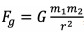# Practical Exercise 4: Gravitational Force

Practical Exercise: Calculate the magnitude of the gravitational force between two grains, which are perfect spheres with a radius of 0.1 m and a density of 1,300 kg m–3, located at a distance of 1 m from each other.

Answer: The gravitational force is calculated using the equation,

where G = 6,67 ∙ 10−11 m3  · kg−1 · s−2. First, we have to calculate the weight of the grains. It is a sphere whose volume is calculated according to the equation.

he result after substitution V = 4,2 ∙ 10−3 m3. The weight is calculated m = ρ V. The grain weight is 5,5 kg.

The gravitational force is then Fg = 2,0 ∙ 10−9 N.

Practical Exercise: Now, imagine that one grain is 10 meters in size and the grains are 1,000 meters apart. Calculate the magnitude of the gravitational force between the grains.

Answer: V = 4,2 ∙ 103 m3 , m = 5,5 ∙ 106 kg, Fg = 2,0 ∙ 10−3 N.

Practical Exercise: What would be the magnitude of the gravitational force if one grain had a diameter of 10 km, the other 0.1 meters and they were 15 km apart?

Answer: V1 = 4,2 ∙ 1012 m3 , m1 = 5,5 ∙ 1015 kg, V2 = 4,2 ∙ 10−3 m3 , m2 = 5,5 kg,

Fg = 9,0 ∙ 10−3 N.